# I.E. Irodov Solutions on The First Law of Thermodynamics-Heat Capacity

Thermodynamics in physics is a branch that deals with heat, work and temperature, and their relation to energy, radiation and physical properties of matter. The first law of thermodynamics relates to heat, internal energy, and work. Expert faculty at BYJU’S prepared solutions for the problems covered in I.E. Irodov bookPART TWO. THERMODYNAMICS AND MOLECULAR PHYSICS - 2.2. The First Law of Thermodynamics. Heat Capacity. These solutions will help students to achieve better scores for JEE Main exam and other entrance exams. By referring to I.E. Irodov book solutions, students can achieve higher marks and self analyse their areas of weaknesses and also to help them solve problems of higher difficulty level in an efficient way.

### I.E. Irodov Solutions on The First Law of Thermodynamics-Heat Capacity

1. Demonstrate that the interval energy U of the air in a room is independent of temperature provided the outside pressure p is constant. Calculate U, if p is equal to the normal atmospheric pressure and the room's volume is equal to V = 40 m3.

Solution:

1. Internal energy of air, treating as an ideal gas

$U = \frac{m}{M}C_vT = \frac{m}{M} \frac{R}{\gamma-1}T = \frac{pV}{\gamma-1}$ ....(1)

Using Cv = R/( γ-1), since Cp - Cv = R and Cp / Cv = γ

Thus, at constant pressure U = constant, because the volume of the room is a constant. Putting the value of p = Patm and V in equation (1), we get U = 10 MJ.

2. A thermally insulated vessel containing a gas whose molar mass is equal to M and the ratio of specific heats Cp/Cv = γ moves with a velocity v. Find the gas temperature increment resulting from the sudden stoppage of the vessel.

Solution:

1. From energy conservation

Ui + (1/2)(vM)v2 = Uf

Or ∆U = (1/2)vMv2 ……(1)

But U = v(RT/(γ-1), ∆U = [vR/(γ-1)] ∆T ..(2)

From (1) and (2)

∆T = Mv2(γ-1)/2R

3. Two thermally insulated vessels 1 and 2 are filled with air and connected by a short tube equipped with a valve. The volumes of the vessels, the pressures and temperatures of air in them are known (V1, p1, T1 and V2, p2, T2). Find the air temperature and pressure established after the opening of the valve.

Solution:

1. On opening the valve, the air will flow from the vessel at higher pressure to the vessel at lower pressure till both vessels have the same air pressure. If this air pressure is p, the total volume of the air in the two vessels will be (V1 + V2). Also, if v1 and v2 be the number of moles of air initially in the two vessels, we have

p1V1 = v1RT1 and p2V2 = v2RT2 …….(1)

After the air is mixed up, the total number of moles are (v1 + v2) and the mixture is at temperature T.

p(v1 + v2) = (v1 + v2)RT …….(2)

Let us look at the two portions of air as one single system. Since this system is contained in a thermally insulated vessel, no heat exchange is involved in the process.

Total heat transfer for the combined system =Q = 0

Moreover, this combined system does not perform mechanical work either. The walls of the containers are rigid and there are no pistons etc to be pushed, looking at the total system, A = 0.

Hence, internal energy of the combined system docs not change in the process. Initially energy of the combined system is equal to the sum of internal energies of the two portions of air:

Ui = U1 + U2 = (v1RT1)/(γ-1) + (v2RT2)/ (γ-1) ….(3)

Final internal energy of (n1 + n2) moles of air at temperature T is given by

Uf = (v1 + v2)RT/(γ-1) …..(4)

Therefore, Ui – Uf

Final temperature and final pressure: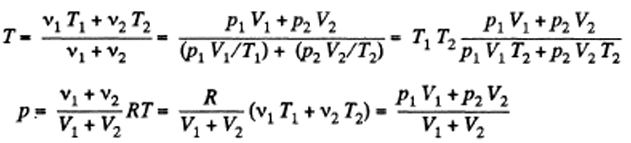4. Gaseous hydrogen contained initially under standard conditions in a sealed vessel of volume V = 5.0 l was cooled by ΔT = 55 K. Find how much the internal energy of the gas will change and what amount of heat will be lost by the gas.

Solution:

1. By the first law of thermodynamics, Q = ΔU + A

Here A = 0, as the volume remains constant, Q = ∆U = (vR/(γ-1))∆T

From gas law: poV = vRTo

∆U = (pov∆T/To(γ-1)) = -0.25 kJ

5. What amount of heat is to be transferred to nitrogen in the isobaric heating process for that gas to perform the work A = 2.0 J?

Solution:

1. By the first law of thermodynamics, Q = ΔU + A

But ΔU = p∆V/( γ-1) = A/ (γ-1)

[p is constant]

Q = A/ (γ-1) + A = γA/( γ-1) = 7 J

6. As a result of the isobaric heating by ΔT = 72 K one mole of a certain ideal gas obtains an amount of heat Q = 1.60 kJ. Find the work performed by the gas, the increment of its internal energy, and the value of γ = Cp/Cv.

Solution:

1. Under isobaric process: A = pΔV = RΔT

[As v = 1]

A = 0.6 kJ

By the first law of thermodynamics, Q = ΔU + A

ΔU = Q – A = Q – RΔT = 1 kJ

Again increment in internal energy = ΔU = R ΔT/( γ-1)

For v = 1

Q – RΔT = RΔT/( γ-1)

Or γ = Q/(Q-RΔT) = 1.6

7. Two moles of a certain ideal gas at a temperature T0 = 300 K were cooled isochorically so that the gas pressure reduced n = 2.0 times. Then, as a result of the isobaric process, the gas expanded till its temperature got back to the initial value. Find the total amount of heat absorbed by the gas in this process.

Solution:

1. Let v = 2 moles of the gas. In the first phase, under isochoric process, A1 = 0, therefore from gas law if pressure is reduced n times so that temperature i.e. new temperature becomes T0/n.

From first law of thermodynamic: Q1 = ΔU1 = vRΔT/( γ-1)

$= \frac{vR}{\gamma- 1}(\frac{T_0}{n}-T_0)$

$= \frac{vRT_0(1-n)}{n(\gamma-1)}latex]$

During the second phase (under isobaric process)

A2 = pΔv = vRΔT

From first law of thermodynamic: Q2 = ΔU2 + A2= vRΔT/( γ-1) + γRΔT

= $\frac{vRT_o(n-1)\gamma}{n(\gamma-1}$

The total amount of heat absorbed: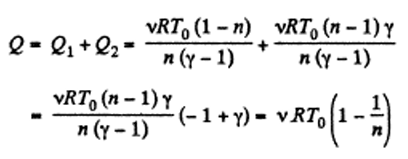8. Calculate the value of γ = Cp/Cv for a gaseous mixture consisting of v1 = 2.0 moles of oxygen and v2 = 3.0 moles of carbon dioxide. The gases are assumed to be ideal.

Solution:

1. Total number of moles of the mixture = v = v1 + v2

At a certain temperature, U = U1 + U2 or vCv = v1Cv1 + v2Cv2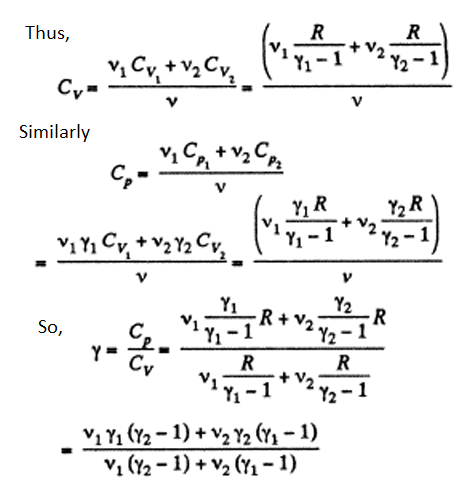9. Find the specific heat capacities cv and cp for a gaseous mixture consisting of 7.0 g of nitrogen and 20 g of argon. The gases are assumed to be ideal.

Solution:

1.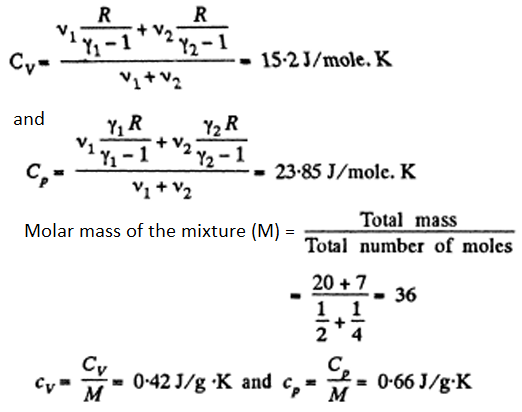10. One mole of a certain ideal gas is contained under a weightless piston of a vertical cylinder at a temperature T. The space over the piston opens into the atmosphere. What work has to be performed in order to increase isothermally the g as volume under the piston it times by slowly raising the piston? The friction of the piston against the cylinder walls is negligibly small.

Solution:

1. Let 5 be the area of the piston and F be the force exerted by the external agent Then, F + pS p0S at an arbitrary instant of time. Here p is the pressure at the instant the volume is V. (Initially the pressure inside is po)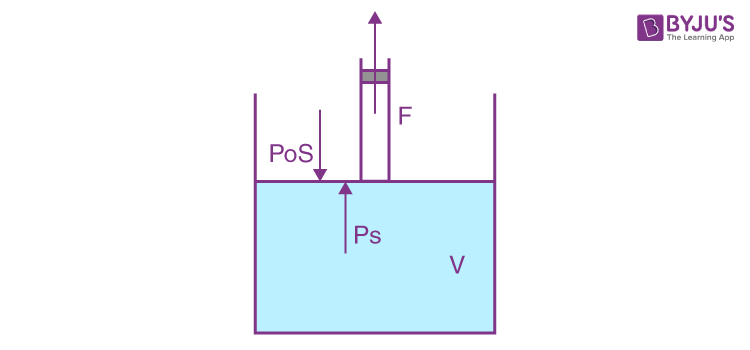= (η - 1)poVo - nRT ln η

= (η - 1)vRT - vRT ln η

= vRT(η – 1 – ln η)

= RT(η – 1 – ln η)

[for v = 1 mole]

11. A piston can freely move inside a horizontal cylinder closed from both ends. Initially, the piston separates the inside space of the cylinder into two equal parts each of volume Vo, in which an ideal gas is contained under the same pressure Po and at the same temperature. What work has to be performed in order to increase isothermally the volume of one part of gas η times compared to that of the other by slowly moving the piston?

Solution:

1. Let the agent move the piston to the right by x. In equilibrium position, p1S + Fagent = p2S or Fagent = (p2 – p1)S

Work done by the agent in an infinitesmal change dx is

Fagent dx = (p2 – p1)S dx = (p2 – p1)dV

By applying pV = constant, for the two parts,

P1(V0 + Sx) = p0V0 and P2(V0 - Sx) = p0V0

So, $p_2-p_1 = \frac{p_0V_02Sx}{V_0^2-S^2x^2}$

or $p_2-p_1 = \frac{2p_0V_0V}{V_0^2-V^2}$

where Sx = V

When the volume of the left end is η times the volume of the right end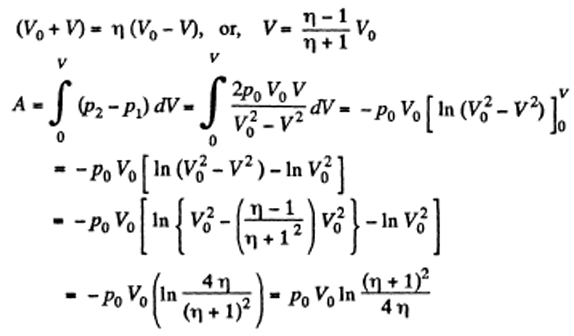12. Three moles of an ideal gas being initially at a temperature To = 273K were isothermally expanded n = 5.0 times its initial volume and then isochorically heated so that the pressure in the final state became equal to that in the initial state. The total amount of heat transferred to the gas during the process equals Q = 80 kJ. Find the ratio γ = Cp/ Cv for this gas.

Solution:

1. In the isothermal process, heat transfer to the gas is given by

$Q_1 = vRT_o ln\frac{V_2}{V_1}=vRT_oln\ \eta$

[for η = V2/V1 = p1/p2]

In the isochoric process, A = 0

Thus, heat transfer to the gas is given by

Q2 = ∆U = vCv ∆T = [vR/( γ-1)] ∆T

[for Cv = R/( γ-1)]

But p2/p1 = To/T or T = To η (for η = p1/p2)

Or ∆T = ηTo – To = (η – 1)To

So, Q2 = [vR/( γ-1)] [η – 1]To

Thus, net heat transfer to the gas

Q = vRTo ln η + vR/( γ-1) (η – 1)To

Q/vRTo = ln η + (η-1)/ ( γ-1)

Or Q/vRTo – ln η = (η-1)/( γ-1)

γ = 1 + (η-1)/[(Q/vRT0 - ln η] = 1.4

13. Draw the approximate plots of isochoric, isobaric, isothermal, and adiabatic processes for the case of an ideal gas, using the following variables: (a) p, T; (b) V, T.

Solution:

1. (a) For ideal gas law: p = (vR/V)T = kT

For isochoric process, obviously k = constant, thus p = kT, represents a straight line passing through the origin and its slope becomes k.

For isobaric process p = constant, thus on p - T curve, it is a horizontal straight line parallel to T - axis, if T is along horizontal (or x - axis)

For isothermal process, T = constant, thus on p - T curve, it represents a vertical straight line if T is taken along horizontal (or x - axis)

For adiabatic process Tγp(1-γ) = constant

After differentiating, we have

(1-γ)p dp T γ + γp1- γ T γ-1 dT = 0

Or dp/dT = [γ/( γ-1)] p/T

The approximate plots of isochoric, isobaric, isothermal, and adiabatic processes are drawn in the answer sheet.

(b) As p is not considered as variable, we have from ideal gas law

V = (vR/p) T = k'T

On V - T co-ordinate system let us, take T along x - axis. For isochoric process V = constant, thus k' = constant and V = k'T obviously represents a straight line passing through the origin of the co-ordinate system and k' is its slope.

For isothermal process T = constant. Thus, on the stated co- ordinate system it represents a straight line parallel to the V - axis.

For adiabatic process, TV γ-1 = constant

After differentiating, we get

(1-γ)Vγ-2 dV T + V γ-1 dT = 0

Or dV/dT =- [1/( γ-1)] V/T

The approximate plots of isochoric, isobaric, isothermal and adiabatic processes are drawn in the answer sheet.

14. One mole of oxygen being initially at a temperature T0 = 290 K is adiabatically compressed to increase its pressure η = 10.0 times. Find: (a) the gas temperature after the compression; (b) the work that has been performed on the gas.

Solution:

1. According to T - p relation in adiabatic process, Tγ = kpγ-1 (where k = constant)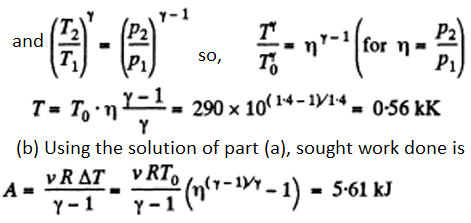15. A certain mass of nitrogen was compressed η = 5.0 times (in terms of volume), first adiabatically, and then isothermally. In both cases the initial state of the gas was the same. Find the ratio of the respective works expended in each compression.

Solution:

1. Let (po, Vo, To) be the initial state of the gas.

But TV γ-1=constant

∆T = To γ-1 – 1)

Thus, Aadia = -vR[To γ-1 – 1)]/(γ-1)

Also, we know Aiso = vRTo ln(1/η) = -vRTo ln (η)

Therefore,

Aadia/ Aiso = [η γ-1 – 1]/[( γ-1) ln η] = 1.4

16. A heat-conducting piston can freely move inside a closed thermally insulated cylinder with an ideal gas. In equilibrium the piston divides the cylinder into two equal parts, the gas temperature being equal to To. The piston is slowly displaced. Find the gas temperature as a function of the ratio η of the volumes of the greater and smaller sections. The adiabatic exponent of the gas is equal to γ.

Solution:

1. Since here the piston is conducting and it is moved slowly the temperature on the two sides increases and maintained at the same value. Elementary work done by the agent = Work done in compression - Work done in expansion

i.e. dA - p2 dV - p1 dV = (p2 - P1) dV

where p1 and p2 are pressures at any instant of the gas on expansion and compression side respectively.

From the gas law, p1(V0 + Sx) = vRT and p2(V0 - Sx) = vRT, for each section (x is the displacement of the piston towards section 2)

So, $p_2-p_1 = vRT \frac{2Sx}{V_0^2-S^2x^2}$

Use Sx = V, then

$dA = vRT \frac{2V}{V_0^2-V^2}dV$

Also, from the first law of thermodynamics

dA = -dU = -2v(R/(γ-1)) dT (as dQ = 0)

So, work done on the gas = -dA = 2v(R/(γ-1)) dT

Thus, 2v(R/(γ-1)) dT = vRT(2VdV)/(V02 – V2)

dT/T = (γ-1)[VdV/(V02 – V2)]

V0 + V = η(V0 + V) or V = (η-1)/( η+1) Vo

On integrating above equation, we have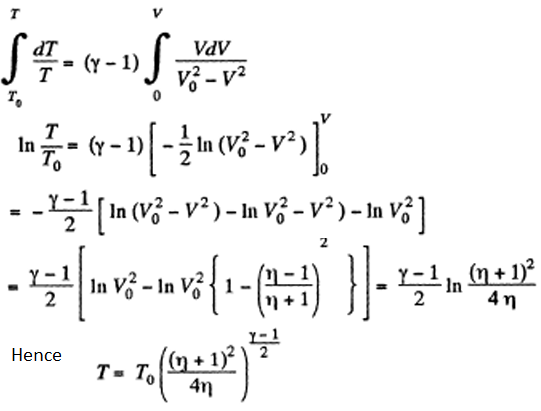17. Find the rate v with which helium flows out of a thermally insulated vessel into vacuum through a small hole. The flow rate of the gas inside the vessel is assumed to be negligible under these conditions. The temperature of helium in the vessel is T = 1,000 K.

Solution:

1. From energy conservation as in the derivation of Bernoulli theorem it reads

p/ρ + (1/2)v2 + gz + u + Qd = constant ......(1)

In the equation (1) u is the internal energy per unit mass and in this case is the thermal energy per unit mass of the gas. As the gas vessel is thermally insulated Qd = 0, also in our case.

Just inside the vessel, u = CvT/M = RT/M(γ-1). Also p/ρ = RT/M.

Inside the vessel v = 0. Just outside p = 0, and u = 0.

In general, gz is not very significant for gases.

Thus applying equation (1) just inside and outside the hole, we get

(1/2)v2 = p/ρ + u

= RT/M + RT/M(γ-1) = γ RT/M(γ-1)

Hence, v2 = 2 γRT/M(γ-1) or v = 3.22 km/s

18. The volume of one mole of an ideal gas with the adiabatic exponent γ is varied according to the law V = a/T, where "a" is a constant. Find the amount of heat obtained by the gas in this process if the gas temperature increased by ΔT.

Solution:

1. The differential work done by the gas

dA = pdV = vRT2/a (-a/T2)dT = -vRdT

(as pV = vRT and V = a/T)

$A = -\int_T^{T+ \Delta T}vRdT = -vR \Delta T$

So, From the First law of thermodynamics

Q = ∆U + A = vR/( γ-1) ∆T - vR∆T

= vR∆T (2- γ)/( γ-1) = R∆T (2- γ)/( γ-1)

(for v = 1 mole)

19. Demonstrate that the process in which the work performed by an ideal gas is proportional to the corresponding increment of its internal energy is described by the equation pVn = const, where n is a constant.

Solution:

1. Here A α U or dA = aU

(where a is proportionality constant)

or, pdV = (avRdT)/(γ-1) ......(1)

From ideal gas law, pV= vRT, on differentiating

pdV + Vdp = v RdT ......(2)

From (1) and (2)

pdV = a/(γ-1) [pdV + Vdp]

Let k = a/(γ-1) = another constant

so, pdV(k - 1) + kVdp = 0

or pdV(k - 1)/k + Vdp = 0

or pdVn + Vdp = 0

where (k - 1)/k = n = ratio

Dividing both sides by pV

n dV/V + dp/p = 0

On integrating, n ln V + ln p = ln C

where C= constant

or ln(pVn) = ln C or pVn = C = constant

20. Find the molar heat capacity of an ideal gas in a polytropic process pVn = const, if the adiabatic exponent of the gas is equal to γ. At what values of the polytropic constant n will the heat capacity of the gas be negative?

Solution:

1. In the polytropic process work done by the gas

$A = \frac{vR(T_i-T_f)}{n-1}$

(where Ti and Tf are initial and final temperature of the gas like in adiabatic process)

$\Delta U = \frac{vR}{\gamma-1}(T_i-T_f)$

By the first law of thermodynamics, Q = ΔU + A

$=\frac{vR}{\gamma-1}(T_i-T_f) + \frac{vR}{n-1}(T_i-T_f)$

$= \frac{vR(n- \gamma)}{(n-1)(\gamma-1)} \Delta T$

According to definition of molar heat capacity when number of moles v = 1 and ΔT = 1 then Q = Molar heat capacity.

$C_n = \frac{R(n-\gamma)}{(n-1)(\gamma-1)} < 0$ for 1 < n < γ.